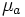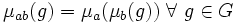# Variety of groups with operators

## Definition

Let$M$ be a monoid. The variety of$M$-groups, or variety of groups with operator monoid$M$, is defined as follows. An algebra in this variety is a group$G$, along with a specified action of$M$ on$G$ by endomorphisms. More precisely, an algebra in this variety has the following operator domain:

1. A binary operation for the group multiplication, a unary operation for the group inverse map, and a constant operation for the identity element of the group. These three operations satisfy the universal identities needed to form a group.
2. For each element of$M$, a unary operation, subject to three kinds of universal identities: two, to ensure that the action is monoidal, and one, to ensure that each element acts by endomorphisms.

Thus, an algebra of this variety is a group$G$, with a binary operation$*$, a unary operation${}^{-1}$, a constant operation$e$, and unary operations$\mu_a$ for every$a \in M$, such that the following are satisfied:

• Associativity:$(g * h) * k = g * (h * k) \ forall \ g,h,k \in G$
• Identity element:$g * e = e * g = g \ \forall \ g,h,k, \in G$
• Inverse element:$g * g^{-1} = g^{-1} * g = e \ \forall \ g \in G$
• Endomorphism property for each$a \in M$:$\mu_a(g * h) = \mu_a(g) * \mu_a(h) \ \forall \ g \in G$
• Composition property for each$a, b \in M$:$\mu_{ab}(g) = \mu_a(\mu_b(g)) \ \forall \ g \in G$
• Identity property (the subscript$e$ denotes the identity element of$M$):$\mu_e(g) = g \ \forall \ g \in G$

In the special case where$M$ is a group, the action of$M$ on$G$ is by automorphisms.